# Draw Shapes

Draw rectangles, lines, polygons, or circles on images

•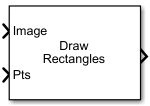Libraries:
Computer Vision Toolbox / Text & Graphics

## Description

The Draw Shapes block draws rectangles, lines, polygons, or circles on images by overwriting the pixel values. As a result, the shapes are drawn on the output image. The block uses Bresenham's line drawing algorithm to draw lines, polygons, and rectangles and Bresenham's circle drawing algorithm to draw circles. The output signal is of the same size and data type as the input signal fed to the Image, R, G, and B ports.

You can set the shape fill or border color via the input port or by manually entering the values using the parameters. Use the color parameters to specify the appearance of the rectangles, lines, polygons, or circles.

## Ports

### Input

expand all

Input image, specified as either an M-by-N matrix of intensity values or an M-by-N-by-3 matrix of color values with 3 color planes.

When the input is a video stream, the block processes it frame by frame.

Data Types: `single` | `double` | `int8` | `int16` | `int32` | `int64` | `uint8` | `uint16` | `uint32` | `uint64` | `Boolean` | `fixed point`

Red color channel of the image or video frame, specified as an M-by-N matrix. The R, G, and B ports must have the same dimensions and data type.

#### Dependencies

To enable this port, set the Image signal parameter to `Separate color signals`.

Data Types: `single` | `double` | `int8` | `int16` | `int32` | `int64` | `uint8` | `uint16` | `uint32` | `uint64` | `Boolean` | `fixed point`

Green color channel of the input image or video frame, specified as an M-by-N matrix. The R, G, and B ports must have the same dimensions and data type.

#### Dependencies

To enable this port, set the Image signal parameter to `Separate color signals`.

Data Types: `single` | `double` | `int8` | `int16` | `int32` | `int64` | `uint8` | `uint16` | `uint32` | `uint64` | `Boolean` | `fixed point`

Blue color channel of the input image or video frame, specified as an M-by-N matrix. The R, G, and B ports must have the same dimensions and data type.

#### Dependencies

To enable this port, set the Image signal parameter to `Separate color signals`.

Data Types: `single` | `double` | `int8` | `int16` | `int32` | `int64` | `uint8` | `uint16` | `uint32` | `uint64` | `Boolean` | `fixed point`

Coordinates of the input shapes to be drawn on the input. The format of the points depends on the type of shape you specify with the Shape parameter.

• `Rectangles`M-by-4 matrix of the form [x y width height]. M is the number of rectangles.

ShapeInput to the Pts PortDrawn Shape
Single RectangleFour-element row vector in the format
`[x y width height]`, where:
• `x` and `y` are the coordinates of the upper-left corner of the rectangle.

• `width` and `height` parameters are the width and height of the rectangle, in pixels. The values of `width` and `height` must be greater than 0.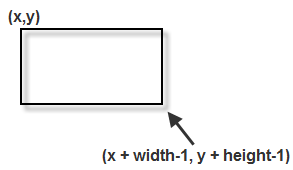M Rectangles

M-by-4 matrix in the format

`$\left[\begin{array}{cccc}{x}_{1}& {y}_{1}& widt{h}_{1}& heigh{t}_{1}\\ {x}_{2}& {y}_{2}& widt{h}_{2}& heigh{t}_{2}\\ ⋮& ⋮& ⋮& ⋮\\ {x}_{M}& {y}_{M}& widt{h}_{M}& heigh{t}_{M}\end{array}\right]$`

,where each row of the matrix corresponds to a different rectangle and is of the same form as the vector for a single rectangle.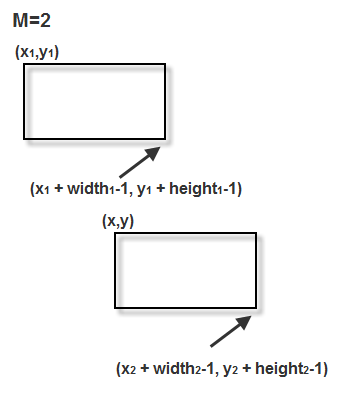• `Lines`M-by-2L matrix of the form [x1, y1, x2, y2,…,xL, yL]. M is the number of polylines.

ShapeInput to the Pts PortDrawn Shape
Single LineFour-element row vector in the format ```[x1 y1 x2 y2]```, where:
• `x1` and `y1` are the coordinates of the beginning of the line.

• `x2` and `y2` are the coordinates of the end of the line.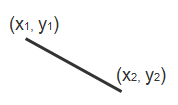M Lines

M-by-4 matrix in the format

`$\left[\begin{array}{cccc}{x}_{11}& {y}_{11}& {x}_{12}& {y}_{12}\\ {x}_{21}& {y}_{21}& {x}_{22}& {y}_{22}\\ ⋮& ⋮& ⋮& ⋮\\ {x}_{M1}& {y}_{M1}& {x}_{M2}& {y}_{M2}\end{array}\right]$`

,where each row of the matrix corresponds to a different line and is of the same form as the vector for a single line.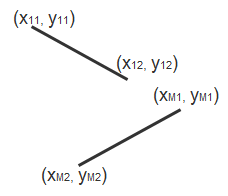Single Polyline with (L-1) SegmentsVector of size 2L, where L is the number of vertices, in the format, ```[x1, y1, x2, y2, ..., xL, yL]```, where
• x1 and y1 are the coordinates of the beginning of the first line segment.

• x2 and y2 are the coordinates of the end of the first line segment and the beginning of the second line segment.

• xL and yL are the coordinates of the end of the (L-1)th line segment.

The polyline always contains (L-1) number of segments because the first and last vertex points do not connect.

The block produces an error message when the number of columns is less than two or not a multiple of two.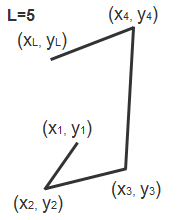M Polylines with (L-1) Segments

M-by-2L matrix in the format

`$\left[\begin{array}{ccccccc}{x}_{11}& {y}_{11}& {x}_{12}& {y}_{12}& \cdots & {x}_{1L}& {y}_{1L}\\ {x}_{21}& {y}_{21}& {x}_{22}& {y}_{22}& \cdots & {x}_{2L}& {y}_{2L}\\ ⋮& ⋮& ⋮& ⋮& \ddots & ⋮& ⋮\\ {x}_{M1}& {y}_{M1}& {x}_{M2}& {y}_{M2}& \cdots & {x}_{ML}& {y}_{ML}\end{array}\right]$`

, where each row of the matrix corresponds to a different polyline and is of the same form as the vector for a single polyline. When one of the polylines contains less than (L–1) number of segments, fill the matrix by repeating the coordinates of the last vertex.

The block produces an error message if the number of columns is less than two or not a multiple of two.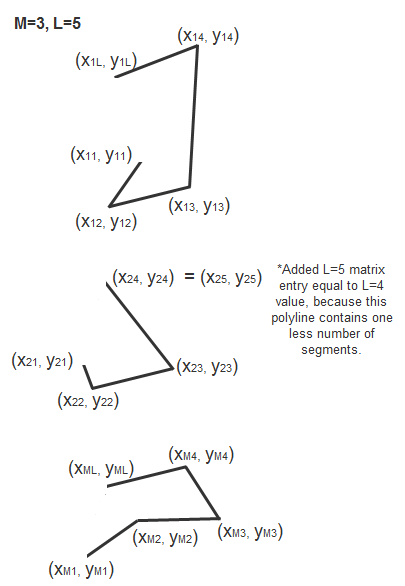• `Polygons`M-by-2L matrix of the form [x1, y1, x2, y2,…,xL, yL]. M is the number of polygons. The points are specified in consecutive order to form a polygon.

ShapeInput to the Pts PortDrawn Shape
Single Polygon with L line segmentsRow vector of size 2L, where L is the number of vertices, in the format ```[x1 y1 x2 y2 ... xL yL]```, where:
• x1 and y1 are the coordinates of the beginning of the first line segment.

• x2 and y2 are the coordinates of the end of the first line segment and the beginning of the second line segment.

• xL and yL are the coordinates of the end of the (L-1)th line segment and the beginning of the Lth line segment.

The block connects ```[x1 y1]``` to ```[xL yL]``` to complete the polygon. The block produces an error if the number of columns is less than two or not a multiple of two.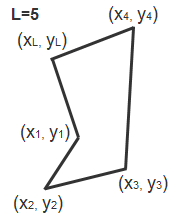M Polygons with the largest number of line segments in any line being L

M-by-2L matrix in the format

`$\left[\begin{array}{ccccccc}{x}_{11}& {y}_{11}& {x}_{12}& {y}_{12}& \cdots & {x}_{1L}& {y}_{1L}\\ {x}_{21}& {y}_{21}& {x}_{22}& {y}_{22}& \cdots & {x}_{2L}& {y}_{2L}\\ ⋮& ⋮& ⋮& ⋮& \ddots & ⋮& ⋮\\ {x}_{M1}& {y}_{M1}& {x}_{M2}& {y}_{M2}& \cdots & {x}_{ML}& {y}_{ML}\end{array}\right]$`

, where each row of the matrix corresponds to a different polygon and is of the same form as the vector for a single polygon.

When one of the polygons contains less than L number of segments, fill the matrix by repeating the coordinates of the last vertex.

The block produces an error message if the number of columns is less than two or not a multiple of two.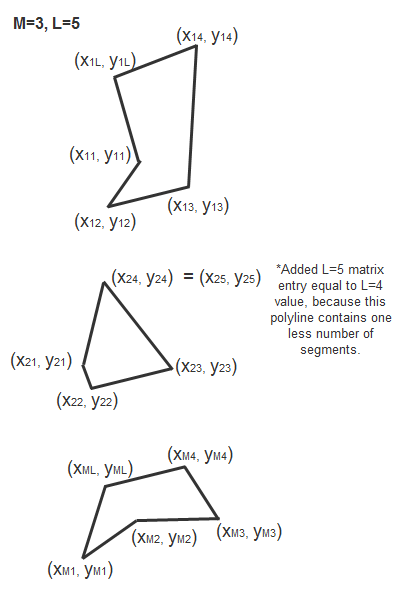• `Circles`M-by-3 matrix of the form [x y radius]. M is the number of circles.

ShapeInput to the Pts PortDrawn Shape
Single CircleThree-element row vector in the format
`[x y radius]`, where:
• `x` and `y` are coordinates for the center of the circle.

• `radius` is the radius of the circle, which must be greater than 0.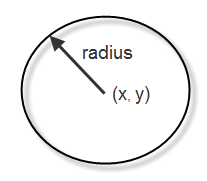M Circles

M-by-3 matrix in the format

`$\left[\begin{array}{ccc}{x}_{1}& {y}_{1}& radiu{s}_{1}\\ {x}_{2}& {y}_{2}& radiu{s}_{2}\\ ⋮& ⋮& ⋮\\ {x}_{M}& {y}_{M}& radiu{s}_{M}\end{array}\right]$`

, where each row of the matrix corresponds to a different circle and is of the same form as the vector for a single circle.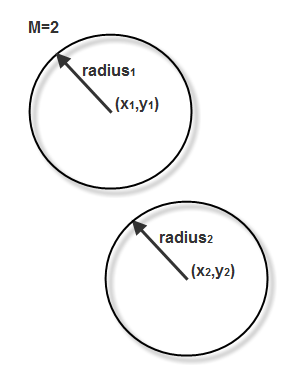#### Dependencies

• Double-precision floating point is only supported if the input to the Image or R, G, and B ports is floating point.

• Single-precision floating point is only supported if the input to the Image or R, G, and B ports is floating point.

Data Types: `single` | `double` | `int8` | `int16` | `int32` | `uint8` | `uint16` | `uint32`

Region of interest, specified as a four-element vector of the form [x y width height]. The region defines a rectangular area to draw shapes. The first two elements represent the one-based coordinates of the upper-left corner of the area. The remaining two elements represent the width and height of the area.

#### Dependencies

To enable this port, set the Draw shapes in parameter to `Specify region of interest via port`.

Data Types: `single` | `double` | `int8` | `int16` | `int32` | `int64` | `uint8` | `uint16` | `uint32` | `uint64` | `Boolean` | `fixed point`

Color of the shape, specified as either a 3-element vector or an M-by-3 matrix. M is the number of shapes and three denotes the number of color planes. You can specify different color for each of the M shapes, or specify one color for all shapes.

#### Dependencies

To enable this port, set the Border color source or Fill color source parameter to ```Input port```.

Data Types: `single` | `double` | `int8` | `int16` | `int32` | `int64` | `uint8` | `uint16` | `uint32` | `uint64` | `Boolean` | `fixed point`

### Output

expand all

Output image, returned as an M-by-N matrix or M-by-N-by-3 array. The output image is of the same dimension as the input image.

Data Types: `single` | `double` | `int8` | `int16` | `int32` | `int64` | `uint8` | `uint16` | `uint32` | `uint64` | `Boolean` | `fixed point` | `enumerated` | `bus`

## Parameters

expand all

Main Tab

One or more shapes to draw on the image, specified as one of the following:

• `Rectangles` — Draw one or more rectangles on the input image or the video frame using the specified shape coordinates.

• `Lines` — Draw one or more lines on the input image or the video frame using the specified shape coordinates.

• `Polygons` — Draw one or more polygons on the input image or the video frame using the specified shape coordinates.

• `Circles` — Draw one or more circles on the input image or the video frame using the specified shape coordinates.

Select Fill shapes to enable the color parameters to fill shapes.

Note

If you are generating the code and selecting the Fill shapes check box, the word length of the block input(s) cannot exceed 16 bits.

Specify the line width for the shapes to be drawn, as a positive scalar.

Specify the input source for the fill color value. Select Fill color source as one of the following:

• `Specify via dialog` — To specify the color value by using Fill color parameter.

• `Input port` — To specify the color value by using Clr port.

#### Dependencies

To enable this parameter, select Fill shapes.

Specify the shape fill color as either `Black`, `White` or `User-specified value`. The `User-specified value` can be scalar or a 3-element vector. Use a scalar to specify an intensity value and 3-element vector to specify RGB color value.

Color Value(s) for Filling One Shape or Multiple Shapes with the Same ColorColor Value(s) for Filling Multiple Shapes with Unique Colors
Scalar intensity valueR-element vector, where R is the number of shapes.
3-element RGB vector R-by-3 matrix, where 3 is the number of color planes and R is the number of shapes.

For each value in the Color Value(s) parameter, enter a number between the minimum and maximum values that can be represented by the data type of the input image. If you enter a value outside this range, the block produces an error message.

#### Dependencies

To enable this parameter, select the Fill shapes parameter.

Specify the input source for the border color value. Select Border color source as one of the following:

• `Specify via dialog` — To specify the color value by using Border color parameter.

• `Input port` — To specify the color value by using Clr port.

Specify the border color of shape as either `Black`, `White` or `User-specified value`. The `User-specified value` can be scalar or a 3-element vector. Use a scalar to specify an intensity value and 3-element vector to specify RGB color value.

Color Value(s) for Drawing One Shape or Multiple Shapes with the Same ColorColor Value(s) for Drawing Multiple Shapes with Unique Colors
Scalar intensity valueR-element vector, where R is the number of shapes.
3-element RGB vector R-by-3 matrix, where three is the number of color planes and R is the number of shapes.

For each value in the Color Value(s) parameter, enter a number between the minimum and maximum values that can be represented by the data type of the input image. If you enter a value outside this range, the block produces an error message.

Specify color value to draw the border or fill the shape as a scalar or 3-element vector. Use a scalar to specify intensity value and a 3-element vector for RGB color value.

Tunable: Yes

#### Dependencies

To enable this parameter set the Border color or Fill color parameter to ```User-specified value```.

Specify the opacity of the color as a positive value in the range [0, 1]. `0` is transparent and `1` is opaque.

Opacity Factor value for Filling One Shape or Multiple Shapes with the Same ColorOpacity Factor value for Filling Multiple Shapes with Unique Colors
Scalar intensity valueR-element vector where R is the number of shapes

#### Dependencies

To enable this parameter, select the Fill shapes parameter.

Performs smoothing on the line, polygon, or circle.

#### Dependencies

• To enable this parameter, set the Shape parameter to either `Lines`, `Polygons`, or `Circles`.

Specify the extent of the region to draw the specified shape. Select the value as one of the following:

• `Entire image` — To draw the shape on the entire image.

• `Specify region of interest via port` — To draw the shape within an ROI specified by the input ROI port.

Specify the number of input ports to use for specifying the input image. Select the value as one of the following:

• `One multidimensional signal` — To specify intensity or color image using a single port. The block enables the Image port for a M-by-N-by-3 color image, or a M-by-N intensity image.

• `Separate color signals` — To specify red, green, and, blue color planes of the input color image. The block enables the R,G, and, B input ports. Each port accepts the M-by-N plane of an RGB color image.

Data Types Tab

For details on the fixed-point block parameters, see Specify Fixed-Point Attributes for Blocks.

## Block Characteristics

 Data Types `Boolean` | `double` | `fixed point` | `integer` | `single` Multidimensional Signals `yes` Variable-Size Signals `yes`

## Version History

Introduced before R2006a